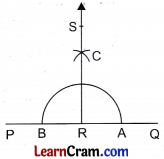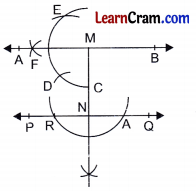# DAV Class 6 Maths Chapter 14 Worksheet 4 Solutions

The DAV Class 6 Maths Book Solutions and DAV Class 6 Maths Chapter 14 Worksheet 4 Solutions of Constructions offer comprehensive answers to textbook questions.

## DAV Class 6 Maths Ch 14 WS 4 Solutions

Question 1.
Draw a line PQuestion Take a point R on it. Draw a line RS perpendicular to PQ using compasses and ruler.Step 1: Draw a line PQ and take any point R on it.
Step 2: Draw a semi-circle with centre R which meets PQ at A and B.
Step 3: Take A and B as centres with radius more than half of arc AB, draw two arcs intersecting each other at C.
Step 4: Join RC and produce to S. Then RS is the required perpendicular to PQ.

Question 2.
Draw a line L. Take a point A outside the line l. From A, draw a line m perpendicular to line l.Step 1: Draw a line l and take a point A outside the line.
Step 2: Taking A as centre and. with suitable radius, draw an arc such that it intersects the line at B and C.
Step 3: Take B and C as centres and with suitable radius, draw two arcs intersecting each other at D.
Step 4: Join AD which intersects line l at P.
Then AP is required perpendicular from A to line l.Question 3.
Draw a line segment XY of length 8 cm. At X and Y, draw perpendiculars to XY using compasses and ruler. Are the lines parallel to each other?Step 1: Draw XY = 8 cm.
Step 2: Take X as centre and suitable radius draw an arc which intersects the line XY at N.
Step 3: Take N as centre and radius equal to the radius taken in step 2, draw an arc intersecting this arc at Question
Step 4: Draw another arc with centre Q and same radius X N GY intersecting the previous arc at P.
Step 5: Draw two arcs with centres P and Q and suitable radius intersecting each other at R.
Step 6: Join XR and produce to A.
Step 7: Draw a perpendicular line YB at Y with the same procedure.
Then XA is parallel to YB.

Question 4.
Draw a line PQuestion Take a point M outside it. Through M, draw a line parallel to PQ using compasses and ruler.